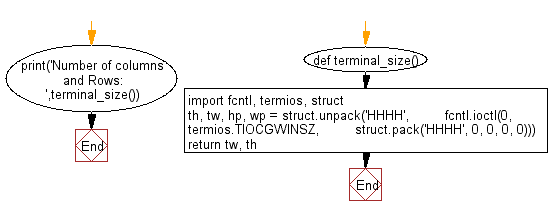﻿ Python: Get height and the width of console window - w3resource# Python: Get height and the width of console window

## Python Basic: Exercise-56 with Solution

Write a Python program to get height and the width of console window.

Sample Solution:-

Python Code:

``````def terminal_size():
import fcntl, termios, struct
th, tw, hp, wp = struct.unpack('HHHH',
fcntl.ioctl(0, termios.TIOCGWINSZ,
struct.pack('HHHH', 0, 0, 0, 0)))
return tw, th

print('Number of columns and Rows: ',terminal_size())
```
```

Sample Output:

```Number of columns and Rows:  (110, 21)
```

Flowchart:Python Code Editor:

Have another way to solve this solution? Contribute your code (and comments) through Disqus.

What is the difficulty level of this exercise?

Test your Programming skills with w3resource's quiz.

﻿

```>>> a = ['j', 'a', 'k', 'd', 'c']